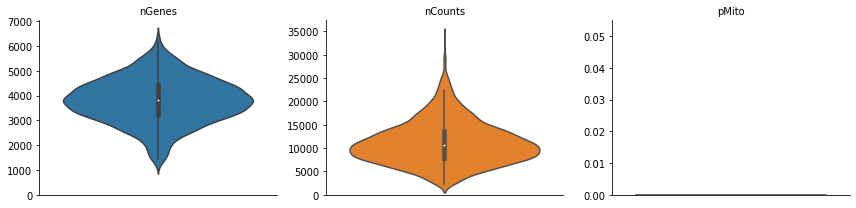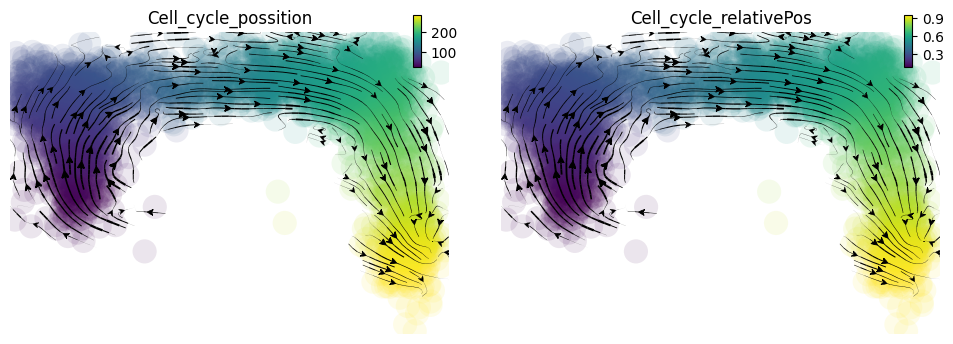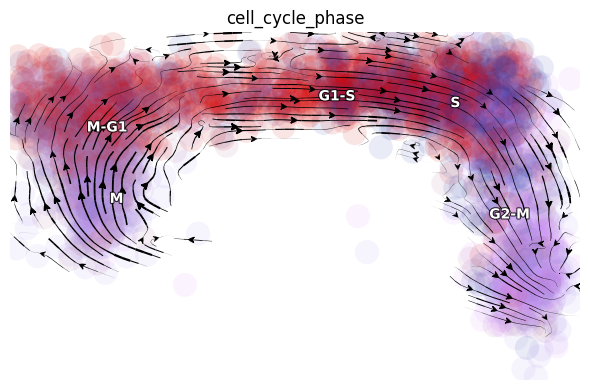# scEU-seq cell cycle¶

This tutorial uses the cell cycle dataset from Battich, et al (2020). This tutorial is the first one of the two tutorials for demonstrating how dynamo can use used to analyze the scEU-seq data. Please refer the organoid tutorial for details on how to analyze the organoid dataset.

Recently Battich and colleague reported scEU-seq as a method to sequence mRNA labeled with 5-ethynyl-uridine (EU) in single cells. By developing a very creative labeling strategy (personally this is my favorite labeling strategy from all available labeling based scRNA-seq papers!) they are able to estimate of RNA transcription and degradation rates in single cell across time.

They applied scEU-seq and the labeling strategy to study the transcription and degradation rates for both the cell cycle and differentiation processes. Similar to what has been discovered in bulk studies, they find the transcription rates are highly dynamic while the degration rate tend to be more stable across different time points. Furthermore, by quantifying the correlation between the transcription rate and degration rates across time, they reveal major regulatory strategies which have distinct consequences for controlling the dynamic range and precision of gene expression.

For both of the cell cycle and the organoid systems, the authors use kinetics and a mixture of pulse and chase experiment to label the cells. I had a lot fun to analyze this complicate dataset. But for the sake of simplicity, here I am going to only use the fraction of kinetics experiment for demonstrating how dynamo can be used to estimate labeling based RNA velocity and to reconstruct vector field function.

# get the latest pypi version # to get the latest version on github and other installations approaches, see: # https://dynamo-release.readthedocs.io/en/latest/ten_minutes_to_dynamo.html#how-to-install !pip install dynamo-release --upgrade --quiet
In :
import warnings
warnings.filterwarnings('ignore')

import dynamo as dyn
import anndata
import pandas as pd
import numpy as np
import scipy.sparse

from anndata import AnnData
from scipy.sparse import csr_matrix

dyn.get_all_dependencies_version()

package dynamo-release pre-commit cvxopt trimap numdifftools colorcet python-igraph pynndescent hdbscan loompy matplotlib networkx numba numpy pandas scikit-learn scipy seaborn setuptools statsmodels tqdm umap-learn
version 1.0.0 2.11.1 1.2.6 1.4.3.dev1 0.9.39 2.0.6 0.9.0 0.5.2 0.8.27 3.0.6 3.4.3 2.5.1 0.53.1 1.19.5 1.3.3 0.23.2 1.7.0 0.11.1 54.2.0 0.12.2 4.58.0 0.5.1

Let us first take a look at number of cells collected at labeling time point for either the pulse or chase experiment.

In :
rpe1 = dyn.sample_data.scEU_seq_rpe1()

|-----> Downloading scEU_seq data

In :
dyn.convert2float(rpe1, ['Cell_cycle_possition', 'Cell_cycle_relativePos'])

In :
rpe1

Out:
AnnData object with n_obs × n_vars = 5422 × 11848
obs: 'Plate_Id', 'Condition_Id', 'Well_Id', 'RFP_log10_corrected', 'GFP_log10_corrected', 'Cell_cycle_possition', 'Cell_cycle_relativePos', 'exp_type', 'time'
var: 'Gene_Id'
layers: 'sl', 'su', 'ul', 'uu'
In :
rpe1.obs.exp_type.value_counts()

Out:
Pulse    3058
Chase    2364
Name: exp_type, dtype: int64
In :
rpe1[rpe1.obs.exp_type=='Chase', :].obs.time.value_counts()

Out:
120     541
0       460
60      436
240     391
360     334
dmso    202
Name: time, dtype: int64
In :
rpe1[rpe1.obs.exp_type=='Pulse', :].obs.time.value_counts()

Out:
30      574
45      564
15      442
120     408
60      405
180     400
dmso    265
Name: time, dtype: int64

## Subset the kinetics experimetn data¶

For the sake of simplicity, I am going to just focus on the kinetics experiment dataset analysis.

In :
rpe1_kinetics = rpe1[rpe1.obs.exp_type=='Pulse', :]
rpe1_kinetics.obs['time'] = rpe1_kinetics.obs['time'].astype(str)
rpe1_kinetics.obs.loc[rpe1_kinetics.obs['time'] == 'dmso', 'time'] = -1
rpe1_kinetics.obs['time'] = rpe1_kinetics.obs['time'].astype(float)
rpe1_kinetics = rpe1_kinetics[rpe1_kinetics.obs.time != -1, :]

rpe1_kinetics.layers['new'], rpe1_kinetics.layers['total'] = rpe1_kinetics.layers['ul'] + rpe1_kinetics.layers['sl'], rpe1_kinetics.layers['su'] + rpe1_kinetics.layers['sl'] + rpe1_kinetics.layers['uu'] + rpe1_kinetics.layers['ul']

del rpe1_kinetics.layers['uu'], rpe1_kinetics.layers['ul'], rpe1_kinetics.layers['su'], rpe1_kinetics.layers['sl']

In :
rpe1_kinetics

Out:
AnnData object with n_obs × n_vars = 2793 × 11848
obs: 'Plate_Id', 'Condition_Id', 'Well_Id', 'RFP_log10_corrected', 'GFP_log10_corrected', 'Cell_cycle_possition', 'Cell_cycle_relativePos', 'exp_type', 'time'
var: 'Gene_Id'
layers: 'new', 'total'

## Perform a typical dynamo analysis¶

A typical analysis in dynamo includes:

1. the preprocessing procedure;
2. kinetic estimation and velocity calculation;
3. dimension reduction;
4. high dimension velocity projection;
5. vector field reconstruction



Note that in the preprocess stages, we calculate some basic statistics, the number of genes, total UMI counts and percentage of mitochondrian UMIs in each cell so we can visualize them via dyn.pl.basic_stats. Moreover, if the adata.var_name is not official gene names but ensemble gene ids, dynamo will try to automatically convert those gene ids into more readable official gene names.

In :
dyn.pl.basic_stats(rpe1_kinetics)In :
rpe1_genes = ['UNG', 'PCNA', 'PLK1', 'HPRT1']

In :
rpe1_kinetics.obs.time

Out:
Cell_02365     15.0
Cell_02366     30.0
Cell_02367     30.0
Cell_02368     30.0
Cell_02369     45.0
...
Cell_05418    120.0
Cell_05419    120.0
Cell_05420    180.0
Cell_05421    180.0
Cell_05422    180.0
Name: time, Length: 2793, dtype: float64
In :
rpe1_kinetics.obs.time  = rpe1_kinetics.obs.time.astype('float')
rpe1_kinetics.obs.time = rpe1_kinetics.obs.time/60 # convert minutes to hours

In :
rpe1_kinetics.obs.time.value_counts()

Out:
0.50    574
0.75    564
0.25    442
2.00    408
1.00    405
3.00    400
Name: time, dtype: int64

## Estimate time-resolved RNA velocity¶

There are some very non-trivial consideration between estimating time-resolved RNA velocity and estimating transcription/degration kinetics rates. The following code provides the right strategy for time-resolved RNA velocity analysis. Wait for our final publication for more in-depth discussion in this interesting topic!

In :
dyn.tl.recipe_kin_data(adata=rpe1_kinetics,
keep_filtered_genes=True,
keep_raw_layers=True,
del_2nd_moments=False,
tkey='time',
)

|-----> keep_filtered_cells_key is None. Using default value from DynamoAdataConfig: keep_filtered_cells_key=False
|-----> apply Monocole recipe to adata...
|-----> convert ensemble name to official gene name
Dynamo is converting those names to official gene names.
|-----> Storing myGene name info into local cache db: mygene_cache.sqlite.

[ Future queries will be cached in "/lab/solexa_weissman/xqiu/proj/Aristotle/dynamo-tutorials/mygene_cache.sqlite" ]
querying 1-1000...done. [ from cache ]
querying 1001-2000...done. [ from cache ]
querying 2001-3000...done. [ from cache ]
querying 3001-4000...done. [ from cache ]
querying 4001-5000...done. [ from cache ]
querying 5001-6000...done. [ from cache ]
querying 6001-7000...done. [ from cache ]
querying 7001-8000...done. [ from cache ]
querying 8001-9000...done. [ from cache ]
querying 9001-10000...done. [ from cache ]
querying 10001-11000...done. [ from cache ]
querying 11001-11848...done. [ from cache ]
Finished.
1 input query terms found dup hits:
[('ENSG00000229425', 2)]
33 input query terms found no hit:
['ENSG00000116957', 'ENSG00000130723', 'ENSG00000168078', 'ENSG00000189144', 'ENSG00000205664', 'ENS
Pass "returnall=True" to return complete lists of duplicate or missing query terms.

|-----> Subsetting adata object and removing Nan columns from adata when converting gene names.
|-----> <insert> pp to uns in AnnData Object.
|-----------> <insert> has_splicing to uns['pp'] in AnnData Object.
|-----------> <insert> has_labling to uns['pp'] in AnnData Object.
|-----------> <insert> splicing_labeling to uns['pp'] in AnnData Object.
|-----------> <insert> has_protein to uns['pp'] in AnnData Object.
|-----> ensure all cell and variable names unique.
|-----> ensure all data in different layers in csr sparse matrix format.
|-----> ensure all labeling data properly collapased
|-----> detected experiment type: kin
|-----------> <insert> tkey to uns['pp'] in AnnData Object.
|-----------> <insert> experiment_type to uns['pp'] in AnnData Object.
|-----> filtering cells...
|-----> <insert> pass_basic_filter to obs in AnnData Object.
|-----> 2793 cells passed basic filters.
|-----> filtering gene...
|-----> <insert> pass_basic_filter to var in AnnData Object.
|-----> 10494 genes passed basic filters.
|-----> calculating size factor...
|-----> selecting genes in layer: X, sort method: SVR...
|-----> <insert> frac to var in AnnData Object.
|-----> size factor normalizing the data, followed by log1p transformation.
|-----> applying PCA ...
|-----> <insert> pca_fit to uns in AnnData Object.
|-----> <insert> ntr to obs in AnnData Object.
|-----> <insert> ntr to var in AnnData Object.
|-----> cell cycle scoring...
|-----> computing cell phase...
|-----> [cell phase estimation] in progress: 100.0000%
|-----> [cell phase estimation] finished [37.6547s]
|-----> <insert> cell_cycle_phase to obs in AnnData Object.
|-----> <insert> cell_cycle_scores to obsm in AnnData Object.
|-----> [Cell Cycle Scores Estimation] in progress: 100.0000%
|-----> [Cell Cycle Scores Estimation] finished [0.5534s]
|-----> [recipe_monocle preprocess] in progress: 100.0000%
|-----> [recipe_monocle preprocess] finished [16.8244s]
|-----> calculating first/second moments...
|-----> [moments calculation] in progress: 100.0000%
|-----> [moments calculation] finished [33.0179s]
|-----? Your adata only has labeling data, but NTR_vel is set to be False. Dynamo will reset it to True to enable this analysis.
|-----> experiment type: kin, method: twostep, model: deterministic
Estimate gamma via linear regression of t vs. -ln(1-K): 1000it [00:04, 206.20it/s]
|-----> retrive data for non-linear dimension reduction...
|-----> perform umap...
|-----> [dimension_reduction projection] in progress: 100.0000%
|-----> [dimension_reduction projection] finished [31.8623s]
|-----> incomplete neighbor graph info detected: connectivities and distances do not exist in adata.obsp, indices not in adata.uns.neighbors.
|-----> Neighbor graph is broken, recomputing....
|-----> Start computing neighbor graph...
|-----------> X_data is None, fetching or recomputing...
|-----> fetching X data from layer:None, basis:pca
|-----> method arg is None, choosing methods automatically...
|-----------> method ball_tree selected
|-----> <insert> connectivities to obsp in AnnData Object.
|-----> <insert> distances to obsp in AnnData Object.
|-----> <insert> neighbors to uns in AnnData Object.
|-----> <insert> neighbors.indices to uns in AnnData Object.
|-----> <insert> neighbors.params to uns in AnnData Object.
|-----> 0 genes are removed because of nan velocity values.
|-----> [calculating transition matrix via pearson kernel with sqrt transform.] in progress: 100.0000%
|-----> [calculating transition matrix via pearson kernel with sqrt transform.] finished [5.6145s]
|-----> [projecting velocity vector to low dimensional embedding] in progress: 100.0000%
|-----> [projecting velocity vector to low dimensional embedding] finished [1.3877s]

Out:
AnnData object with n_obs × n_vars = 2793 × 11385
obs: 'Plate_Id', 'Condition_Id', 'Well_Id', 'RFP_log10_corrected', 'GFP_log10_corrected', 'Cell_cycle_possition', 'Cell_cycle_relativePos', 'exp_type', 'time', 'nGenes', 'nCounts', 'pMito', 'pass_basic_filter', 'total_Size_Factor', 'initial_total_cell_size', 'Size_Factor', 'initial_cell_size', 'new_Size_Factor', 'initial_new_cell_size', 'ntr', 'cell_cycle_phase'
var: 'Gene_Id', 'nCells', 'nCounts', 'query', 'scopes', '_id', '_score', 'symbol', 'notfound', 'pass_basic_filter', 'score', 'log_cv', 'log_m', 'use_for_pca', 'frac', 'ntr', 'alpha', 'a', 'b', 'alpha_a', 'alpha_i', 'beta', 'p_half_life', 'gamma', 'half_life', 'cost', 'logLL', 'gamma_k', 'gamma_r2', 'mean_R2', 'use_for_dynamics', 'use_for_transition'
uns: 'pp', 'velocyto_SVR', 'PCs', 'explained_variance_ratio_', 'pca_mean', 'pca_fit', 'feature_selection', 'cell_phase_genes', 'dynamics', 'neighbors', 'umap_fit', 'grid_velocity_umap'
obsm: 'X_pca', 'X', 'cell_cycle_scores', 'X_umap', 'velocity_umap'
layers: 'new', 'total', 'X_total', 'X_new', 'M_t', 'M_tt', 'M_n', 'M_tn', 'M_nn', 'velocity_N', 'velocity_T', 'cell_wise_alpha'
obsp: 'moments_con', 'distances', 'connectivities', 'pearson_transition_matrix'
In :
rpe1_kinetics

Out:
AnnData object with n_obs × n_vars = 2793 × 11385
obs: 'Plate_Id', 'Condition_Id', 'Well_Id', 'RFP_log10_corrected', 'GFP_log10_corrected', 'Cell_cycle_possition', 'Cell_cycle_relativePos', 'exp_type', 'time', 'nGenes', 'nCounts', 'pMito', 'pass_basic_filter', 'total_Size_Factor', 'initial_total_cell_size', 'Size_Factor', 'initial_cell_size', 'new_Size_Factor', 'initial_new_cell_size', 'ntr', 'cell_cycle_phase'
var: 'Gene_Id', 'nCells', 'nCounts', 'query', 'scopes', '_id', '_score', 'symbol', 'notfound', 'pass_basic_filter', 'score', 'log_cv', 'log_m', 'use_for_pca', 'frac', 'ntr', 'alpha', 'a', 'b', 'alpha_a', 'alpha_i', 'beta', 'p_half_life', 'gamma', 'half_life', 'cost', 'logLL', 'gamma_k', 'gamma_r2', 'mean_R2', 'use_for_dynamics', 'use_for_transition'
uns: 'pp', 'velocyto_SVR', 'PCs', 'explained_variance_ratio_', 'pca_mean', 'pca_fit', 'feature_selection', 'cell_phase_genes', 'dynamics', 'neighbors', 'umap_fit', 'grid_velocity_umap'
obsm: 'X_pca', 'X', 'cell_cycle_scores', 'X_umap', 'velocity_umap'
layers: 'new', 'total', 'X_total', 'X_new', 'M_t', 'M_tt', 'M_n', 'M_tn', 'M_nn', 'velocity_N', 'velocity_T', 'cell_wise_alpha'
obsp: 'moments_con', 'distances', 'connectivities', 'pearson_transition_matrix'

## Visualize velocity streamlines¶

When we project our estimated transcriptomic RNA velocity to the space formed by the $log_{10}(fluorescence), Geminin -GFP$ and $log_{10}(fluorescence), Cdt1-RFP$ (Geminin and Cdt1 are two markers of different cell cycle stages), we can a nice transition from early cell cycle stage to late cell cycle stage.

In :
def streamline(adata):

rpe1_kinetics.obsm['X_RFP_GFP'] = rpe1_kinetics.obs.loc[:, ['RFP_log10_corrected', 'GFP_log10_corrected']].values.astype('float')
streamline(rpe1_kinetics)

|-----> retrive data for non-linear dimension reduction...
|-----? adata already have basis umap. dimension reduction umap will be skipped!
set enforce=True to re-performing dimension reduction.
|-----> [dimension_reduction projection] in progress: 100.0000%
|-----> [dimension_reduction projection] finished [0.0023s]
|-----> 0 genes are removed because of nan velocity values.
|-----> [calculating transition matrix via pearson kernel with sqrt transform.] in progress: 100.0000%
|-----> [calculating transition matrix via pearson kernel with sqrt transform.] finished [5.8654s]
|-----> [projecting velocity vector to low dimensional embedding] in progress: 100.0000%
|-----> [projecting velocity vector to low dimensional embedding] finished [1.4523s]Out:
AnnData object with n_obs × n_vars = 2793 × 11385
obs: 'Plate_Id', 'Condition_Id', 'Well_Id', 'RFP_log10_corrected', 'GFP_log10_corrected', 'Cell_cycle_possition', 'Cell_cycle_relativePos', 'exp_type', 'time', 'nGenes', 'nCounts', 'pMito', 'pass_basic_filter', 'total_Size_Factor', 'initial_total_cell_size', 'Size_Factor', 'initial_cell_size', 'new_Size_Factor', 'initial_new_cell_size', 'ntr', 'cell_cycle_phase'
var: 'Gene_Id', 'nCells', 'nCounts', 'query', 'scopes', '_id', '_score', 'symbol', 'notfound', 'pass_basic_filter', 'score', 'log_cv', 'log_m', 'use_for_pca', 'frac', 'ntr', 'alpha', 'a', 'b', 'alpha_a', 'alpha_i', 'beta', 'p_half_life', 'gamma', 'half_life', 'cost', 'logLL', 'gamma_k', 'gamma_r2', 'mean_R2', 'use_for_dynamics', 'use_for_transition'
uns: 'pp', 'velocyto_SVR', 'PCs', 'explained_variance_ratio_', 'pca_mean', 'pca_fit', 'feature_selection', 'cell_phase_genes', 'dynamics', 'neighbors', 'umap_fit', 'grid_velocity_umap', 'grid_velocity_RFP_GFP'
obsm: 'X_pca', 'X', 'cell_cycle_scores', 'X_umap', 'velocity_umap', 'X_RFP_GFP', 'velocity_RFP_GFP'
layers: 'new', 'total', 'X_total', 'X_new', 'M_t', 'M_tt', 'M_n', 'M_tn', 'M_nn', 'velocity_N', 'velocity_T', 'cell_wise_alpha'
obsp: 'moments_con', 'distances', 'connectivities', 'pearson_transition_matrix'

Since dynamo automatically performs the cell cycle staging at the preprocess step with cell-cycle related marker genes using methods from Norman et. al. We can also check whether dynamo's staging makes any sense for this dataset. Interesting, dynamo staging indeed reveals a nice transition from M stage to M-G, to G1-S, to S and finally to G2-M stage. This is awesome!

In :
dyn.pl.streamline_plot(rpe1_kinetics, color=['cell_cycle_phase'], basis='RFP_GFP')

<Figure size 600x400 with 0 Axes>## Animate cell cycle transition¶

In :
dyn.vf.VectorField(rpe1_kinetics, basis='RFP_GFP', map_topography=True, M=50)

|-----> VectorField reconstruction begins...
|-----> Retrieve X and V based on basis: RFP_GFP.
Vector field will be learned in the RFP_GFP space.
|-----> Generating high dimensional grids and convert into a row matrix.
|-----> Learning vector field with method: sparsevfc.
|-----> [SparseVFC] begins...
|-----> Sampling control points based on data velocity magnitude...
|-----> [SparseVFC] in progress: 100.0000%
|-----> [SparseVFC] finished [0.6670s]
|-----> <insert> velocity_RFP_GFP_SparseVFC to obsm in AnnData Object.
|-----> <insert> X_RFP_GFP_SparseVFC to obsm in AnnData Object.
|-----> <insert> VecFld_RFP_GFP to uns in AnnData Object.
|-----> Mapping topography...
|-----> <insert> control_point_RFP_GFP to obs in AnnData Object.
|-----> <insert> inlier_prob_RFP_GFP to obs in AnnData Object.
|-----> <insert> obs_vf_angle_RFP_GFP to obs in AnnData Object.
|-----> [VectorField] in progress: 100.0000%
|-----> [VectorField] finished [2.9857s]

In :
progenitor = rpe1_kinetics.obs_names[rpe1_kinetics.obs.Cell_cycle_relativePos < 0.1]
len(progenitor)

Out:
78
In :
np.random.seed(19491001)

from matplotlib import animation
info_genes = rpe1_kinetics.var_names[rpe1_kinetics.var.use_for_transition]
dyn.pd.fate(rpe1_kinetics, basis='RFP_GFP', init_cells=progenitor, interpolation_num=100,  direction='forward',
inverse_transform=False, average=False)

integration with ivp solver: 100%|██████████| 78/78 [00:06<00:00, 11.40it/s]
uniformly sampling points along a trajectory: 100%|██████████| 78/78 [00:00<00:00, 217.37it/s]

Out:
AnnData object with n_obs × n_vars = 2793 × 11385
obs: 'Plate_Id', 'Condition_Id', 'Well_Id', 'RFP_log10_corrected', 'GFP_log10_corrected', 'Cell_cycle_possition', 'Cell_cycle_relativePos', 'exp_type', 'time', 'nGenes', 'nCounts', 'pMito', 'pass_basic_filter', 'total_Size_Factor', 'initial_total_cell_size', 'Size_Factor', 'initial_cell_size', 'new_Size_Factor', 'initial_new_cell_size', 'ntr', 'cell_cycle_phase', 'control_point_RFP_GFP', 'inlier_prob_RFP_GFP', 'obs_vf_angle_RFP_GFP'
var: 'Gene_Id', 'nCells', 'nCounts', 'query', 'scopes', '_id', '_score', 'symbol', 'notfound', 'pass_basic_filter', 'score', 'log_cv', 'log_m', 'use_for_pca', 'frac', 'ntr', 'alpha', 'a', 'b', 'alpha_a', 'alpha_i', 'beta', 'p_half_life', 'gamma', 'half_life', 'cost', 'logLL', 'gamma_k', 'gamma_r2', 'mean_R2', 'use_for_dynamics', 'use_for_transition'
uns: 'pp', 'velocyto_SVR', 'PCs', 'explained_variance_ratio_', 'pca_mean', 'pca_fit', 'feature_selection', 'cell_phase_genes', 'dynamics', 'neighbors', 'umap_fit', 'grid_velocity_umap', 'grid_velocity_RFP_GFP', 'cell_cycle_phase_colors', 'VecFld_RFP_GFP', 'fate_RFP_GFP'
obsm: 'X_pca', 'X', 'cell_cycle_scores', 'X_umap', 'velocity_umap', 'X_RFP_GFP', 'velocity_RFP_GFP', 'velocity_RFP_GFP_SparseVFC', 'X_RFP_GFP_SparseVFC'
layers: 'new', 'total', 'X_total', 'X_new', 'M_t', 'M_tt', 'M_n', 'M_tn', 'M_nn', 'velocity_N', 'velocity_T', 'cell_wise_alpha'
obsp: 'moments_con', 'distances', 'connectivities', 'pearson_transition_matrix'
In :
%%capture
import matplotlib.pyplot as plt

fig, ax = plt.subplots()
ax = dyn.pl.topography(rpe1_kinetics, basis='RFP_GFP', color='Cell_cycle_relativePos', ax=ax, save_show_or_return='return', fps_basis='RFP_GFP')
ax.set_aspect(0.8)

In :
%%capture
instance = dyn.mv.StreamFuncAnim(adata=rpe1_kinetics, basis='RFP_GFP', color='Cell_cycle_relativePos', ax=ax, fig=fig)

|-----? the number of cell states with fate prediction is more than 50. You may want to lower the max number of cell states to draw via cell_states argument.

In :
import matplotlib
matplotlib.rcParams['animation.embed_limit'] = 2**128 # Ensure all frames will be embedded.

from matplotlib import animation
import numpy as np

anim = animation.FuncAnimation(instance.fig, instance.update, init_func=instance.init_background,
frames=np.arange(100), interval=100, blit=True)
from IPython.core.display import display, HTML
HTML(anim.to_jshtml()) # embedding to jupyter notebook.

Out: